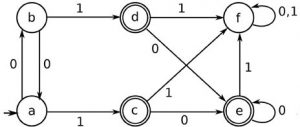# Ittiam Written Test Questions

Round 1(30 mins): Written Test
It was a written round (pen-paper). It started just after the company’s ppt (pre-placement talk). There were a total of 20 Mcqs (with negative marking), 10 from quantitative aptitude and 10 from technical aptitude to be solved in 30 mins.

Quant questions were from time&work, speed-distance(tricky one), few maths quesns viz. related to area of circle, mathematical reasoning (like questions on propositional logic, there exists and for all ->these type of questions can be found in discrete mathematics). Overall it was of average difficulty level.

1) What is the return value of count when the function start() is called with num value of 2017 in the following program:

 `int` `start(``int` `num) ` `{ ` `    ``int` `count = 0; ` `    ``while` `(num > 0) { ` `        ``++count; ` `        ``num = (num - 1) & num; ` `    ``} ` `    ``return` `count; ` `} ` `} `

a)25
b)1
c)7
d)8

Ans) c

2)Global Variables can be shared between :
a)Between both two process and two threads
b)By processes only but not between Threads
c)By Threads only but not between processes

Ans) c

3)You have 3 baskets, one with apples, one with oranges and one with both apples and oranges mixed. Each basket is closed and is labeled with ‘Apples’, ‘Oranges’ and ‘Apples and Oranges’. However, each of these labels is always placed incorrectly. How many fruits would you pick only one fruit from a basket to place the labels correctly on all the baskets?

a)3
b)1
c)4
d)2

Ans) d

4)

 `#include ` ` `  `int` `main(``void``) ` `{ ` `  ``unsigned ``int` `x=10; ` `  ``unsigned ``int` `y=0; ` `  ``while` `(x > 0) ` `  ``{ ` `    ``--x; ` `    ``++y; ` `  ``} ` `  ``printf``(``"%u"``, y); ` `}  `

a)5
b)7
c)10
d)The function does not return anything
Ans) c

5)What is the final state that is been reached after the traversing of the string ‘000100110’:a)b
b)f
c)a
d)e

Ans) b

6)Study the following table and answer the questions based on it.
Expenditures of a Company (in Lakh Rupees) per Annum Over the given Years.

```Year    Item of Expenditure Salary    Fuel and Transport
Bonus    Interest on Loans    Taxes
1998    288    98    3.00    23.4    83
1999    342    112    2.52    32.5    108
2000    324    101    3.84    41.6    74
2001    336    133    3.68    36.4    88
2002    420    142    3.96    49.4    98```

What is the average amount of interest per year which the company had to pay during this period?
a)Rs. 32.43 lakhs
b)Rs. 33.72 lakhs
c)Rs. 34.18 lakhs
d)Rs. 36.66 lakhs
Ans) d

7) If a person walks at 14 km/hr instead of 10 km/hr, he would have walked 20 km more. The actual distance traveled by him is:
a)50 km
b)56 km
c)70 km
d)80 km
Ans) a

8) Which of the following line of code is suitable to start a thread?

 `class` `X ``implements` `Runnable  ` `{  ` `    ``public` `static` `void` `main(String args[])  ` `    ``{ ` `        ``/* Missing code? */` `    ``}  ` `    ``public` `void` `run() {}  ` `} `